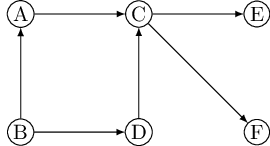# Glossary

## Algo: Topological sorting

Suppose that you need to perform many tasks, but some of them cannot begin until certain others are completed (you have to wake up before you can get out of bed; you have to be out of bed, but not yet dressed, to take a shower; and so on). The question then is, what is a valid order in which to perform the tasks?

Such constraints are conveniently represented by a directed graph in which each task is a node, and there is an edge from $u$ to $v$ if $u$ is a precondition for $v$. In other words, before performing a task, all the tasks pointing to it must be completed. If this graph has a cycle, there is no hope: no ordering can possibly work. If on the other hand the graph is a dag, we would like if possible to linearize (or topologically sort) it, to order the vertices one after the other in such a way that each edge goes from an earlier vertex to a later vertex, so that all precedence constraints are satisfied. In the following figure, for instance, one valid ordering is $B, A, D, C, E, F$. (Can you spot the other three?)What types of dags can be linearized? Simple: All of them. And once again depth-first search tells us exactly how to do it: simply perform tasks in decreasing order of their ${\tt post}$ numbers. After all, the only edges $(u, v)$ in a graph for which ${\tt post}(u)<{\tt post}(v)$ are back edges (recall the table of edge types in DFS entry)—and we have seen that a dag cannot have back edges. Therefore:

Property In a dag, every edge leads to a vertex with a lower ${\tt post}$ number.

This gives us a linear-time algorithm for ordering the nodes of a dag. And, together with our earlier observations, it tells us that three rather different-sounding properties—acyclicity, linearizability, and the absence of back edges during a depth-first search—are in fact one and the same thing.

Since a dag is linearized by decreasing ${\tt post}$ numbers, the vertex with the smallest ${\tt post}$ number comes last in this linearization, and it must be a sink—no outgoing edges. Symmetrically, the one with the highest ${\tt post}$ is a source, a node with no incoming edges.

Property Every dag has at least one source and at least one sink.

The guaranteed existence of a source suggests an alternative approach to linearization:

• Find a source, output it, and delete it from the graph.
• Repeat until the graph is empty.